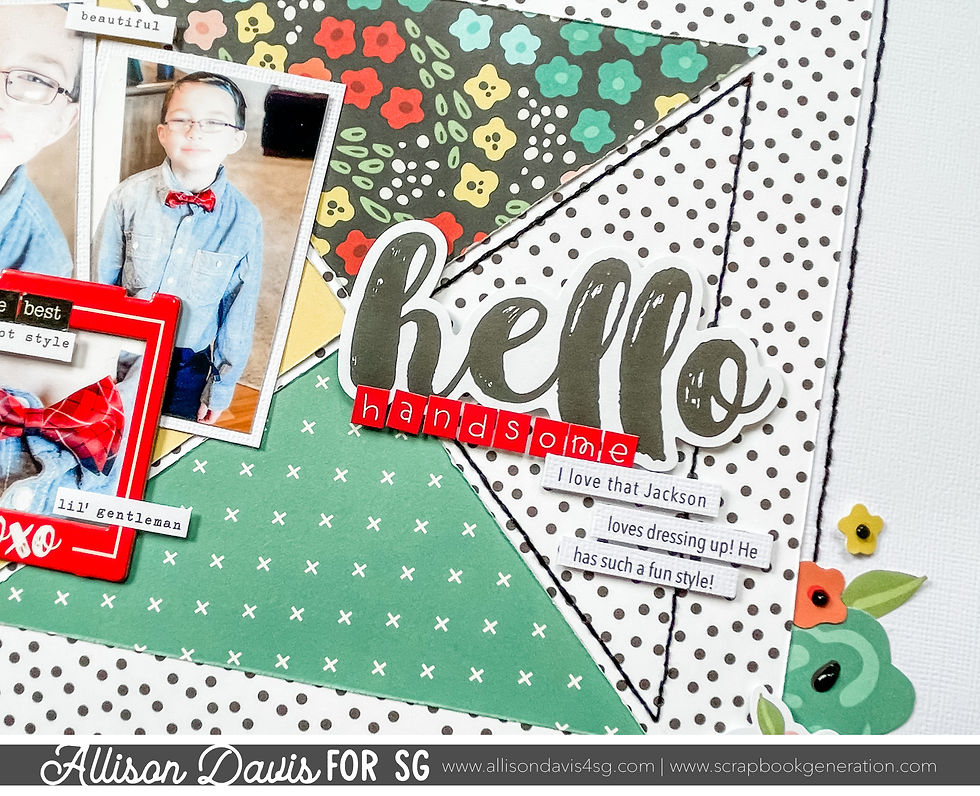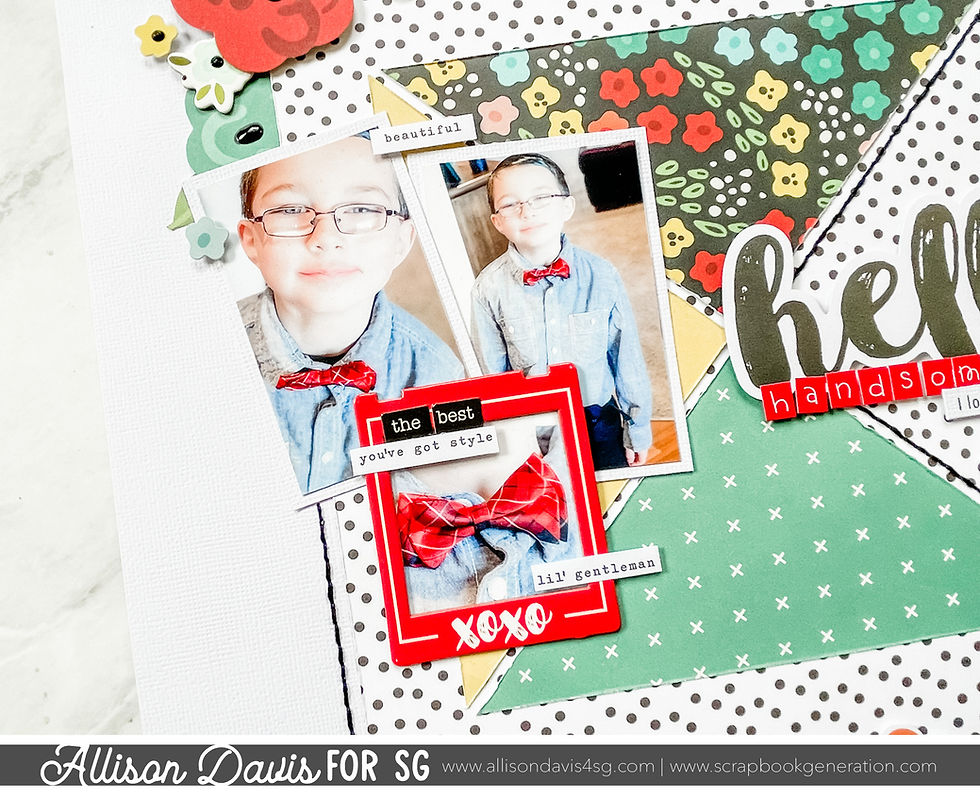top of page
Search

# Scrapbook Design Idea: Creating with Triangles

The layout I'm sharing today is one I had created based a sketch for 6x6" paper pads. It was during on of the Facebook group/classes I hosted a few years ago. You can find the sketches here: Sketches for 6x6 Paper Pads: One-page SketchesOne of my go-to designs to use with 6x6" papers pads is to create a square background out of triangle pieces. What I love about this is that even though it's so simple, there are so many ways you can customize it to look different each time you create it.

On this layout I used three patterned paper triangles and left one open with a stitched border. I also added a larger background piece (not from a 6x6" paper pad) to frame the triangles.You can group two, three, or four triangles together to create square designs. This is a wonderful way to introduce more colors and patterns into designs using squares. And, they work for all sizes of squares from small to large.

Here are a few ideas for creating squares with triangles:

Two triangles - To create squares with two triangles cut a square in half diagonally. So if you want a 6” square made up of two triangles with two different patterned papers, you would start with two 6” squares and cut each one in half diagonally. Then take one triangle from each square and place them together.

Three triangles - To create squares with three triangles cut a square in half diagonally for the largest triangle. For the two smaller triangles you cut two squares in half diagonally just like you did for the first largest triangle, then cut that triangle half sheet in half again, this time from the middle point/corner to the middle of the longest edge. This gives you a square with one large triangle for half the square and two smaller triangles the other half.Four triangles - To create squares with four triangles you will start with four squares of patterned paper. Cut each one in half diagonally. Then cut four of those triangles in half from the middle point/corner to the middle of the longest edge.

A great trick to break that size barrier with 6x6 papers is to use triangles cut from a 6” square and arranging them to create a larger square. The diagonal edge of a triangle cut from a 6” square is 8-1/2” and since those diagonal edges become the outer edge of the square, you end up with an 8-1/2 x 8-1/2” square. Compare that to cutting a triangle piece from a 6” square in half (like the example for 4 triangles) and ending up with a 6” square of triangles. It’s a big difference!To complete the design I framed two opposite corners with flower clusters.Shop all sketches here: Allison Davis Sketches

Want to see more? Find me on...